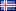ÍslenskaEnglish

27.070
4.564
1.492
2.284

# Lokaverkefni (Meistara)

Vinsamlegast notið þetta auðkenni þegar þið vitnið til verksins eða tengið í það: http://hdl.handle.net/1946/39487

Titill:
•Evaluation of power loss from corona discharge on an ACSR ZEBRA 220 kV conductor
Námsstig:
• Meistara
Höfundur:
Efnisorð:
Útdráttur:
•When designing high voltage transmission lines, power losses are minimized in the design, including corona losses. To date, there are no definitive texts that explain how corona loss is calculated in practice. This thesis will investigate two empirically derived formulas, the Peek corona loss formula and the Peterson corona loss formula. Their derivations are detailed, as well as their working conditions, and how power loss relates to parameters such as spacing between conductors, frequency, operating voltage, and environmental factors. Several methods were used to digitalize an empirical coefficient used in the Peterson formula, known as the phi factor. The process of of digitalizing this factor from an image is detailed. The results are shown using working ranges, where the Peek formula is used to quantify losses above 1.8e_0, where e_0 is the disruptive critical voltage, and the Peterson formula is used to quantify losses in the range of 1.0<e_n/e_0<1.8, where e_n is the line to neutral voltage of the line. The results clearly show a mismatch between the two formulas even at 1.8e_0, which is the boundary where they should meet. Through a detailed review of each formula's derivation, it is found that the Peek empirical formula was obtained by doing a practical experiment while Peterson empirical formula obtained by practical geometric layout of the conductor. It is postulated that this is a reason for the mismatch. The discrepancy is narrowed down to the way Peek calculated the disruptive gradient g_0, which was found to be too high, when comparing to the methods Peterson used to calculate the disruptive critical voltage. Peterson's method included geometric factors such as the exact number of strands, making it more applicable to the cable chosen for this study. The process to derive both formulas was done in Matlab and used a Zebra cable data as an experiment conductor to evaluate the corona loss. A better understanding of corona loss could help people reduce and prevent such losses on high voltage transmission lines.

Samþykkt:
• 25.6.2021
URI:
• http://hdl.handle.net/1946/39487

Skrár
Skráarnafn Stærð AðgangurLýsingSkráartegund
Evaluation of power loss from corona discharge on an ACSR ZEBRA 220 kV Conductor.pdf1.44 MBOpinnHeildartextiPDF